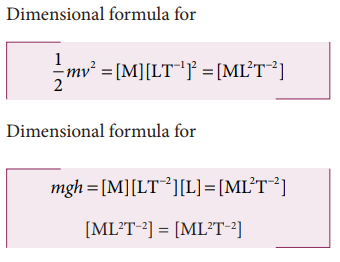Home | | Physics 11th std | Application and Limitations of the Method of Dimensional Analysis

# Application and Limitations of the Method of Dimensional Analysis

Application and Limitations of the Method of Dimensional Analysis

Application and Limitations of the Method of Dimensional Analysis.

This method is used to

i. Convert a physical quantity from one system of units to another.

ii. Check the dimensional correctness of a given physical equation.

iii. Establish relations among various physical quantities.

### i. To convert a physical quantity from one system of units to another

This is based on the fact that the product of the numerical values (n) and its corresponding unit (u) is a constant. i.e, n [u] = constant (or) n1[u1] = n2[u2].

Consider a physical quantity which has dimension ‘a’ in mass, ‘b’ in length and ‘c’ in time. If the fundamental units in one system are M1, L1 and T1 and the other system are M2, L2 and T2 respectively, then we can write, n1 [M1a L1b T1c] = n2 [M2a L2b T2c]

We have thus converted the numerical value of physical quantity from one system of units into the other system.

### (ii) To check the dimensional correctness of a given physical equation

Let us take the equation of motion

v = u + at

Apply dimensional formula on both sides

[LT−1] = [LT−1] + [LT−2] [T]

[LT−1] = [LT−1] + [LT−1]

(Quantities of same dimension only can be added)

We see that the dimensions of both sides are same. Hence the equation is dimensionally correct.

### (iii) To establish the relation among various physical quantities

If the physical quantity Q depends upon the quantities Q1, Q2 and Q3 ie. Q is proportional to Q1, Q2 and Q3.

Then,

Q α Q1a Q2b Q3c

Q = k Q1a Q2b Q3c

where k is a dimensionless constant. When the dimensional formula of Q, Q1, Q2 and Q3 are substituted, then according to the principle of homogeneity, the powers of M, L, T are made equal on both sides of the equation. From this, we get the values of a, b, c

## Limitations of Dimensional analysis

This method gives no information about the dimensionless constants in the formula like 1, 2, ……..π,e, etc.

This method cannot decide whether the given quantity is a vector or a scalar.

This method is not suitable to derive relations involving trigonometric, exponential and logarithmic functions.

It cannot be applied to an equation involving more than three physical quantities.

It can only check on whether a physical relation is dimensionally correct but not the correctness of the  relation.  For  example  using dimensional analysis, s = ut + 1/3 at2 is dimensionally correct whereas the correct relation is s = ut + 1/2 at2.

## Solved Example Problems

### (i) To convert a physical quantity from one system of units to another

Example 1.12

Convert 76 cm of mercury pressure into Nm−2 using the method of dimensions.

Solution

In cgs system 76 cm of mercury pressure = 76 × 13.6 × 980 dyne cm−2

The dimensional formula of pressure P is [ML−1T−2]Example 1.13

If the value of universal gravitational constant in SI is 6.6x10−11 Nm2 kg−2, then find its value in CGS System?

Solution

Let GSI be the gravitational constant in the SI system and Gcgs in the cgs system. ThenThe dimensional formula for G is M−1 L3T −2### (ii) To check the dimensional correctness of a given physical equation

Example 1.14

Check the correctness of the equationusing dimensional analysis method

SolutionBoth sides are dimensionally the same, hence the equationsis dimensionally correct.

### (iii) To establish the relation among various physical quantities

Example 1.15

Obtain an expression for the time period T of a simple pendulum. The time period T depends on (i) mass ‘m’ of the bob (ii) length ‘l’ of the pendulum and (iii) acceleration due to gravity g at the place where the pendulum is suspended. (Constant k = 2π) i.e

SolutionHere k is the dimensionless constant. Rewriting the above equation with dimensionsComparing the powers of M, L and T on both sides, a=0, b+c=0, -2c=1

Solving for a,b and c a = 0, b = 1/2, and c = −1/2

From the above equation T = k. m0  l1/2 g−1/2Example 1.16

The force F acting on a body moving in a circular path depends on mass of the body (m), velocity (v) and radius (r) of the circular path. Obtain the expression for the force by dimensional analysis method. (Take the value of k=1)where k is a dimensionless constant of proportionality. Rewriting above equation in terms of dimensions and taking k = 1, we haveComparing the powers of M, L and T on both sidesFrom the above equation we getTags : Physics , 11th Physics : UNIT 1 : Nature of Physical World and Measurement
Study Material, Lecturing Notes, Assignment, Reference, Wiki description explanation, brief detail
11th Physics : UNIT 1 : Nature of Physical World and Measurement : Application and Limitations of the Method of Dimensional Analysis | Physics